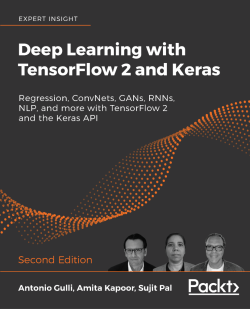# Free eBook: Deep Learning with TensorFlow 2 and Keras - Second EditionBuild machine and deep learning systems with the newly released TensorFlow 2 and Keras for the lab, production, and mobile devices

Antonio Gulli, Amita Kapoor and Sujit Pal, 646 pages, Dec 2019

### Key Features

• Introduces and then uses TensorFlow 2 and Keras right from the start
• Teaches key machine and deep learning techniques
• Understand the fundamentals of deep learning and machine learning through clear explanations and extensive code samples

### Description

Deep Learning with TensorFlow 2 and Keras, Second Edition teaches deep learning techniques alongside TensorFlow (TF) and Keras. The book introduces neural networks with TensorFlow, runs through the main applications, covers two working example apps, and then dives into TF and cloudin production, TF mobile, and using TensorFlow with AutoML.

#### Register now to access this free eBook

Your password must have at least 8 characters, one uppercase, one lowercase and one number.

By signing up, you are confirming you would like to receive occasional emails about special offers and recommendations.

## Chapters

### Chapter 1

Free

#### Neural Network Foundations with TensorFlow 2.0

This chapter provides an intro to neural networks and how to use tf.keras layers in TensorFlow 2 to build simple neural network models. Perceptron, Multilayer Perceptrons, Activation functions, and Dense Networks are discussed. The chapter provides an intuitive introduction to backpropagation.

### Chapter 2

Free

#### TensorFlow 1.x and 2.x

This chapter compares TensorFlow 1.x and TensorFlow 2.0 programming models and how to use TensorFlow 1.x lower-level computational graph APIs, and how to use tf.keras higher-level APIs. New functionalities such as eager computation, Autograph, tf.Datasets, and distributed training are covered.

### Chapter 3

Free

#### Regression

This chapter focuses on the most popular ML technique: regression. You will learn how to use TensorFlow 2.0 estimators to build simple and multiple regression models. You will learn to use logistic regression to solve a multiclass classification problem.

### Chapter 4

Free

#### Convolutional Neural Networks

This chapter introduces CNNs and their applications to image processing. Use TensorFlow 2.0 to build simple CNNs to recognize handwritten characters in the MNIST dataset, and how to classify CIFAR images. Understand how to use pretrained networks such as VGG16 and Inception.

### Chapter 5

Free

This chapter discusses advanced applications of CNNs to image, video, audio, and text processing. Examples of image processing (Transfer Learning, DeepDream), audio processing (WaveNet), and text processing (Sentiment Analysis, Q&A) will be discussed in detail.

### Chapter 6

Free

This chapter focuses on the recently discovered GANs, from the first proposed GAN model, and uses it to forge MNIST characters. It uses deep convolutional GANs to create celebrity images and discusses various GAN architectures like SRGAN, InfoGAN, and CycleGAN and a range of GAN applications.

### Related Titles

Neural Network Projects with Python

Build your Machine Learning portfolio by creating 6 cutting-edge Artificial Intelligence projects using neural networks in Python

Artificial Intelligence with Python

Build real-world Artificial Intelligence applications with Python to intelligently interact with the world around you